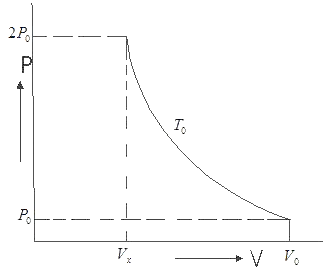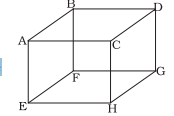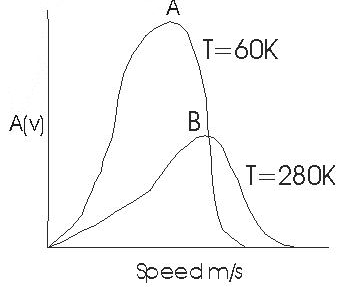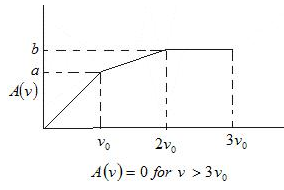# Multiple choice questions on Kinetic theory of gases for Class 11,JEE and NEET

## Multiple choice questions with one or more answer

Question 1
Let A & B are two sample of ideal gases of equal mole .let T be the temperature of both the gas Let $E_A$ and $E_B$ are there total energy respectively .Let $M_A$ and $M_B$ are these respective Molecular Mass .which of these is true
a. $E_A > E_B$
b. $E_A < E_B$
c. $E_A =E_B$
d. none of these

Question 2
An Ideal gas undergoes an state change according to PV diagram. what is the value $V_x$a. $\frac {V_0}{2}$
b. $V_0$
c. $2V_0$
d. $\frac {V_0}{4}$

A container contains $N_2$ gas at T K. The no of moles of gas is $n_0$. Consider it behaves like ideal gas. It rms speed is $v_0$

Question 3
What is the total translational kinetic energy
a. $\frac {3}{2} n_0RT$
b. $\frac {3}{2} RT$
c. $\frac {1}{2} RT$
d. $\frac {1}{2} n_0RT$

Question 4
Suppose the temperature of gas is tripled and $N_2$ molecules dissociate into atom. Then what will be the rms speed of atom.
a. $v_0 \sqrt {6}$
b. $\sqrt {6 v_0}$
c. $v_0 \sqrt {3}$
d. $\sqrt {3 v_0}$

Question 5
if $V_p$ denotes most probable velocity of N2 at T then which of these is correct
a. $V_p : V_0= \sqrt {2} : \sqrt {3}$
b. $V_p : V_0= 1 : 1$
c. $V_p : V_0= 2 : 3$
d. $V_p : V_0= \sqrt {2 }: 3$

## Multiple choice questions with one or more answer

Question 6
An ideal gas undergoes the process describe by equation
$P = P_0 - aV^2$
Where $P_0$ , a are positive constant and V is the volume of one mole of gas
Maximum temperature attainable by gas
a. $\frac {2}{3} (\frac {P_0}{R}) \sqrt { \frac {P_0}{3a}}$
b. $3 P_0 \sqrt {\frac {P_0}{2Ra}}$
c. $(\frac {1}{3R}) \sqrt {\frac {P_0}{3a}}$
d. $\frac {4}{3} (\frac {P_0}{R}) \sqrt { \frac {P_0}{2}}$

Question 7
Let V, Vrms and Vp respectively denotes the mean speed, root mean square speed and most probable speed of the molecule in ideal monatomic gas at absolute temperature T. The mass of the molecule
is m then
a. No molecule can have a speed greater the (2)1/2Vrms
b. No molecule can have a speed less the Vp / (2)1/2
c. Vp < V < Vrms
d. The average kinetic charge of a molecule (3/4 )mVp2

A flask contains Oxygen, Hydrogen & chlorine in the ration of 3:2:1 mixture at 27 °C
Molecular mass of Oxygen = 32
Molecular mass of Hydrogen = 2
Molecular mass of Chlorine = 70.9

Question 8
Find the ratio of average kinetic energy per molecule of Oxygen & Hydrogen
a. 1:2
b. 1:1
c. 1:16
d. 2:1

Question 9
find the ratio of average Kinetic energy per molecule of Hydrogen & chlorine
a. 1:2
b. 1:1
c. 1:16
d. 1:1

Question 10
find the ratio of mean speed of Oxygen, chlorine, Hydrogen
a. 4:1 : (.45)1/2
b. 5:2 :(5)1/2
c. 1: (.45)1/2:4
d. 2 :(.45)1/2 : (1.5)1/2

## Multiple choice questions with one or more answer

Question 11
Suppose a container is evacuated to have just one molecule of a gas in it. Let Va & Vrms represent the Average Speed and rms speed of the molecule
a. Va > Vrms
b. Va < Vrms
c. Va = Vrms
d. Vrms is undefined

Question 12
The velocities of the molecules are v, 2v, 3v, 4v & 5v. The rms speed will be
a. 11v
b. v(11)1/2
c. v
d. 3.3v

Question 13
Equal Number of molecules of hydrogen & Oxygen are contained in a vessel at one atmosphere
pressure. The ratio of the collision frequency of hydrogen molecules to the of Oxygen molecules on the
container
a. 1:4
b. 4:1
c. 1:16
d. 16:1

Question 14
The prefect gases A, B & C having masses m1, m2& m3 at temperature T1, T2 & T3 are mixed without any loses of internal energy of the molecules. The molecules weight of the gases is M1, M2, M3 what
is the final temperature of mixture.
a. [(m1/M1)T1 + (m2/M2)T2 + (m3/M3)T3]/(m1/M1+m2/M2+m3/M3)
b. [(M1/m1)T1 + (M2/m2)T2 + (M3/m3)T3]/(M1/m1+M2/m2+M3/m3)
c. [(m1)T1 + (m2)T2 +(m3)T3]/(m1+m2+m3)
d. [(M1)T1 + (M2)T2 + (M3)T3]/(M1+M2+M3)

Question 15
M moles  of a ideal polyatomic gas(Cv=7R/2)  are in cylinder at temperature T.A heat Q is supplied to the gas. Some M/3 moles of the gas dissociated into atoms while temperature remains constant. Find the correct relation
(a) $3Q=4MRT$
(b) 2Q=3MRT
(c) $Q=4MRT$
(d) $7Q=4MRT$

Question 16
A gas mixture consist of molecules of type A, B, C, D with molecular
masses Ma > Mb > Mc >Md
Two statement are drawn from it
Statement A, Average kinetic energy of four type of gases in the mixture are in the ratio
Ea/1 =Eb/1 =Ec/1 =Ed/1
Statement B, Rms speed of molecules of the four types are in the order if V is the rms speed
VD > VC > VC > VA
which one of following is true
a. Only A correct
b. Only B correct
c. A & B both are correct
d. A & B both are wrong

Assertion and Reason
a. Statement I is true ,statement II is true ,statement II is correct explanation for statement I
b. Statement I is true ,statement II is true ,statement II is not a correct explanation for statement I
c. Statement I is true, Statement II is false
d. Statement I is False, Statement II is True

Question 17
There are two statement
Statement A. Equal volumes of all gases at the same temperature T and pressure P contain an equal no of Molecules
Statement B,.the no of molecules in one mole of any gas is 6.0255 * 1022.
which one of the following is correct
a. A and B both
b. A only
c B only
d. A and B both are incorrect

Question 18
There are two statement about Ideal gases
Statement A The Vrms of gas molecules depends on the mass of the gas molecule and the temperature
Statement B The Vrms is same for all the gases at the same temperature
which one of the following is correct
a. A and B both
b. A only
c. B only
d. A and B both are incorrect

Question 19
STATEMENT 1:The molar specific heat capacity of the ideal gas in isothermal process is infinity
STATEMENT 2:Heat transfer is non zero in the Isothermal process

Question 20
STATEMENT 1:Equation of state for a real gas is (P+a/V2)(V-b)=nRT
STATEMENT 2: Molecular attraction is not negligible and the size of molecules are not negligible in comparison to average separation between them

Question 21
A container has a mixture of 1 mole of oxygen and 2 moles of nitrogen at 330K.The ratio of average rotational kinetic energy per O2 molecule to that per H2 molecule is
a. 2:1
b. 1:2
c. 1:1
d. None of these

Question 22
1 mole of an ideal gas is contained in a cubical volume V, ABCDEFGH at 300 K One face of the cube (EFGH) is made up of a material which totally absorbs any gas molecule incident on it.At any given time
(a) the pressure on EFGH would be zero.
(b) the pressure on all the faces will the equal.
(c) the pressure of EFGH would be double the pressure on ABCD.
(d) the pressure on EFGH would be half that on ABCD.

According the Maxwell's Speed distribution law
$A(v) = 4 \pi (\frac {M}{2\pi RT})^{3/2} v^2 e^{-Mv^2/2RT}$
Where V is molecular speed
M = molar mass of gas
R = gas constant
T = Temperature
A (v) = Probability distribution function
A (v) dv = fraction of the molecules whose speed lie in the internal of width dv speed center on v

Question 23
What is most probable speed
a. $\sqrt {\frac {2RT}{M}}$
b. $\sqrt {\frac {RT}{M}}$
c. $\sqrt {\frac {3RT}{M}}$
d.$\sqrt {\frac {8RT}{M}}$

Question 24
When is of these is true with respect to above paragraph where the integral is from 0 to infinity
a. $\int_{0}^{\infty}A (v) dv = 1$
b. $\int_{0}^{\infty}A (v) dv = 2$
c. $\int_{0}^{\infty}A (v) dv = 1/2$
d. $\int_{0}^{\infty}A (v) dv = 0$

Question 25let A1& A1 are the Area of these two curves which is of the is true
a. A1 = 1     A2 = 1
b. A1 = 1     A2 = 2
c. A1 = 1,     A2 = 1.5
d. None of these

Question 26
What is the formula for Vavg
a. $V_{avg} = \int_{0}^{\infty} vA (v) dv$
b. $V_{avg} = \int_{0}^{\infty} A (v) dv$

c. $V_{avg} = \int_{-\infty}^{\infty} vA (v) dv$
d. $V_{avg} = \int_{-\infty}^{\infty} A (v) dv$

The below figure show a hypothetical speed distribution for a sample of N gas particles.Question 27
What is the relation but a, b & v0
a. $v_0 (2a+3b) = 2$
b. $v_0 (2a- 3b) = 2$
c. $v_0 (a+ b) = 1$
d. $v_0 (a- b) = 1$

Question 28
How much fraction of molecules are in speed range v0 & 3v0
a. $(\frac {v_0}{2}) (3b+a)$
b. $v_0(3b+c)$
c. $(\frac {v_0}{4}) (3b+a)$
d. $(\frac {v_0}{4}) (3b-a)$

Question 29
What is the average speed of the molecules
a. $\frac {6av_0^2 + 20bv_0^2}{6}$
b.$\frac {6av_0^2 - 38bv_0^2}{6}$
c. $3av_0^2 + 2bv_0^2$
d. $3av_0^2 - 2bv_0^2$

Question 30
Column A
A. Average energy of monatomic molecule
B. Average energy of Diatomic molecule
C. Average energy of Polyatomic molecule with no vibration
d. Average energy of Diatomic molecule which vibrate

Column B
U. $3K_BT$
V. $\frac {7}{2} K_BT$
X. $\frac {5}{2} K_BT$
Y. $\frac {3}{2} K_BT$
a. A - V,B - X,C - U,D - Y
b. A - Y,B - X,C - U,D - V
c. A -X,B - Y,C - U,D - V
d. A - Y,B - X,C - V,D - U

Question 31
A vessel of volume V contains a mixture of 1 mole of Hydrogen and 1 mole of Oxygen (both considered as ideal). Let $f_1(v)dv$, denote the fraction of molecules with speed between v and (v + dv) with $f_2 (v)dv$, similarly for oxygen. Then
(a) $f_1(v) + f_2(v) =f(v)$ obeys the Maxwell's distribution law.
(b) $f_1 (v)$, $f_2(v)$ will obey the Maxwell's distribution law separately.
(c) Neither $f_1 (v)$, nor $f_2 (v)$ will obey the Maxwell's distribution law.
(d) $f_2 (v)$ and $f_1 (v)$ will be the same.

Question 32
A gas has five molecules having velocities v,2v,3v,4v,5v.Match the column A to column B
Here Vmean is mean velocity and Vrms is root mean square velocity of the molecules

Column A
P. (Vrms)2
Q. (Vmean)2
R (V2)mean
S. (V2)mean - (Vrms)2

Column B
W. 9v2
X. 11v2
Y. 10v2
Z. 0

a.P-W,Q-X,R-Y,S-Z
b.P-X,Q-W,R-Z,S-Y
c.P-X,Q-W,R-Y,S-Z
d.P-X,Q-W,R-X,S-Z

Question 33
A gas has $v_0$ as rms at temperature $T_0$ and pressure $p_0$.which of the following is correct
a.If the pressure is doubled keeping temperature constant T0 ,rms will remain same
b.if the mass of the gas molecules is tripled,rms will become .58v0
c. if the temperature is increased such that T=4T0,rms will become 2v0
d. None of the above

Question 34
A cubic vessel (with faces horizontal + vertical) contains an ideal gas at NTP. The vessel is being carried by a rocket which is moving at a speed of 200 m/s in vertical direction. The pressure of the gas inside the vessel as observed by us on the ground
(a) remains the same because 200 m/s is very much smaller than $v_{rms}$ of the gas.
(b) remains the same because motion of the vessel as a whole does not affect the relative motion of the gas molecules and the walls.
(c) will increase by a factor equal to $(\frac { v_{rms}^2 + 2500)^2}{v_{rms}^2})$ where $v_{rms}$ was the original mean square velocity of the gas.
(d) will be different on the top wall and bottom wall of the vessel.